# Types of Inheritance in C++

## Types of Inheritance in C++ with Examples

In this article, I am going to discuss Types of inheritance in C++ with Examples. Please read our previous article where we discussed IsA and HasA Relationship in C++ with Examples.

##### Types of Inheritance in C++:

C++ supports five types of inheritances. They are as follows:

1. Single inheritance
2. Multiple inheritance
3. Multilevel inheritance
4. Hierarchical inheritance
5. Hybrid inheritance
##### Single Inheritance in C++:

When a class is derived from a single base class then the inheritance is called single inheritance. For a better understanding, please have a look at the below image.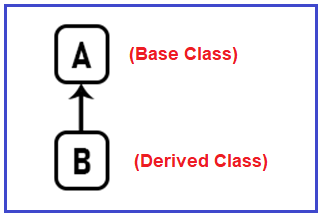If we have a class A that is the Base class and another class B that is the Derived class or child class and B is inheriting from A. Then such type of inheritance is called Single Inheritance in C++. Suppose you have a class Rectangle. From this class, we have written another class that is Cuboid as shown in the below image.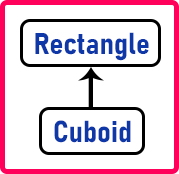This is an example of Single Inheritance. It means a class is inheriting from another class only.

##### Example to understand Single Inheritance in C++:
```#include<iostream>
using namespace std;

//Base class
class Rectangle
{
public:
int length;
int Area ()
{
}
int Perimeter ()
{
return 2 * (length + breadth);
}
};

//Subclass derived from a single base class
class Cuboid:public Rectangle
{
public:
int height;
Cuboid (int l, int b, int h)
{
length = l;
height = h;
}
int Volume ()
{
return length * breadth * height;
}
};

// main function
int main()
{
// Creating object of sub class and
// invoke the methods of base and derived classes
Cuboid c (2, 4, 6);
cout << "Volume is " << c.Volume () << endl;
cout << "Area is " << c.Area () << endl;
cout << "Perimeter is " << c.Perimeter () << endl;
}
```
###### Output: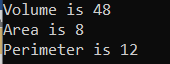##### Hierarchical Inheritance in C++:

When more than one derived class is created from a single base class then it is called Hierarchical inheritance. For a better understanding, please have a look at the below image.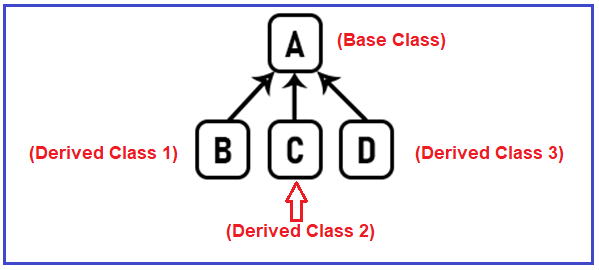Now if you have a class A then from this class there is more than one class inheriting from A i.e. B is inheriting, C is inheriting as well as a D is inheriting. So, when more than one class is inheriting from a Single Base Class, then such a type of inheritance is called Hierarchical Inheritance. Suppose we have a class called Shape. We know that Rectangle, Triangle, Circle, and so on. All these are shapes.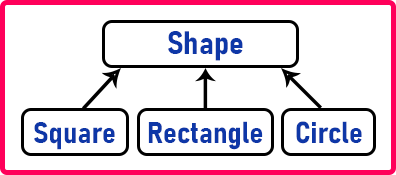Here all the shapes are inheriting from the Shape Base Class. All shapes have their attributes, area, or parameters. We can take an example of the Cars class also. BMW, AUDI, and Innova are inheriting from class the Cars class.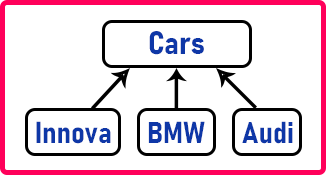In this example, when you have hierarchical inheritance, in this hierarchy the parent class is a generalized class. Innova is a specific car, BMW and Audi are also specific cars but Car is a general term. It is not really existed in the world but we use it as a general term. We can call many 4-wheelers cars so Car is a general term. So, this approach is a generalization approach.

##### Example to Understand Hierarchical Inheritance in C++
```#include <iostream>
using namespace std;

//Base class
class Car
{
public:
Car ()
{
cout << "This is a Car" << endl;
}
};

// First Derived Class
class Innova:public Car
{
public:
Innova ()
{
cout << "This is Innova" << endl;
}
};

//Second Derived Class
class BMW:public Car
{
public:
BMW ()
{
cout << "This is BMW" << endl;
}
};

class Audi:public Car
{
public:
Audi ()
{
cout << "This is Audi" << endl;
}
};

// main function
int main()
{
// Creating object of sub class will
// invoke the constructor of base class.
Innova obj1;
BMW obj2;
Audi obj3;
return 0;
}
```
###### Output: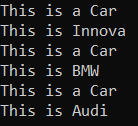##### Multilevel Inheritance in C++:

When a derived class is created from another derived class, then that type of inheritance is called multilevel inheritance. For a better understanding, please have a look at the below image.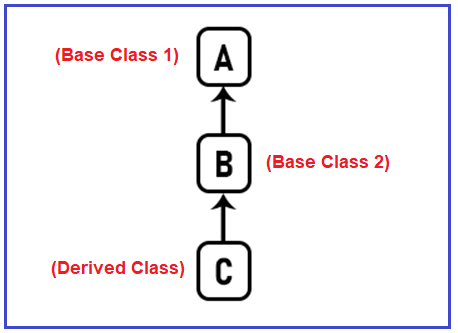If there is a class A and from A, class B is inheriting and from B, class C is inheriting, then such type of inheritance is called Multilevel Inheritance in C++. Suppose we have a class called Point. We know the point is having some coordinates i.e. x and y. And the radius of a point is zero.If we give radius to a point then it becomes a circle.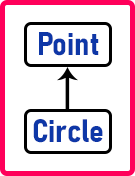If we give height to the circle then it will become a cylinder.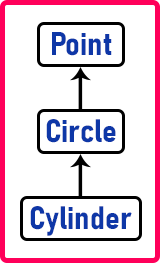So, like this, we can define multi-level classes. So, this is an example of multilevel inheritance.

##### Example to understand Multilevel Inheritance in C++:
```#include<iostream>
using namespace std;

//Base class1
class Point
{
public:
Point ()
{
cout << "Point Constructor Executed" << endl;
}
void GetPoint ()
{

cout << "GetPoint Method Called from Point Class" << endl;
}
};

//Base class 2 Derived from Base class 1
class Circle:Point
{
public:
Circle ()
{
cout << "Circle Constructor Executed" << endl;
}
void GetCircle ()
{
this->GetPoint ();
cout << "GetCircle Method Called from Circle Class" << endl;
}
};

//Dervied Class derived from base class2
class Cylinder:public Circle
{
public:
Cylinder ()
{
cout << "Cylinder Constructor Executed" << endl;
}
void GetCylinder ()
{
cout << "GetCylinder Method Called from Cylinder Class" << endl;
}
};

// main function
int main()
{
// Creating object of Derived class and
// invoke the methods of base classes and derived class
Cylinder obj;
//obj.GetPoint(); //Error
obj.GetCircle ();
obj.GetCylinder ();
}
```
###### Output: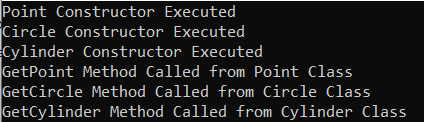##### Multiple Inheritance in C++:

When a derived class is created from more than one base class then such type of inheritance is called multiple inheritances. For a better understanding, please have a look at the below image.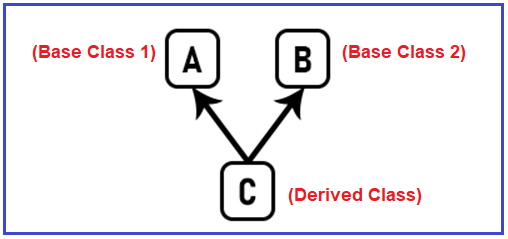If there are classes A and B and from both of them class C is inheriting, then such type of inheritance is called Multiple Inheritance in C++. There is a little different compared to other inheritances. Multiple inheritance means our class can inherit from more than one class in C++. So, it means for one class there can be more than one base class. It is possible in C++ that a class can inherit from more than one class.

If you know a little bit about Java or C#, then it is not allowed in Java or C#. Java and C# don’t have multiple inheritance. So, they have another way of achieving multiple inheritance i.e. using interfaces.

Why does a class need to inherit from multiple classes? Suppose we have a class called SmartPhone. We can use a smartphone like a normal phone as well as a camera. That means there are many things on smartphones. So a smartphone is having a phone, camera, and media player. A lot of things are available but we are showing only two things that is the phone and a camera.##### Example to understand Multiple Inheritance in C++
```#include<iostream>
using namespace std;

//Base class1
class Phone
{
public:
void GetPhoneModel ()
{
cout << "Redmi Note 5 Pro" << endl;
}
};

//Base class2
class Camera
{
public:
{
cout << "24 Mega Pixel Camera" << endl;
}
};

//Subclass derived from a single base class
class SmartPhone:public Phone, public Camera
{
public:
void GetDetails ()
{
cout << "Its a RedMi Smart Phone" << endl;
}
};

// main function
int main()
{
// Creating object of Derived class and
// invoke the methods of base classes and derived class
SmartPhone obj;
obj.GetPhoneModel ();
obj.GetDetails ();
}
```
###### Output: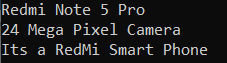##### Hybrid Inheritance in C++:

Hybrid Inheritance is the inheritance that is the combination of any single, hierarchical, and multilevel inheritances. inheritance. For a better understanding, please have a look at the below image.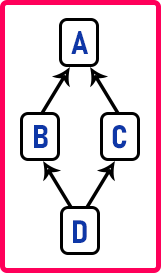There are two subclasses i.e. B and C which are inheriting from class A. Then from B and C there is one more class that is inheriting from B and C which is D. Now this is a combination of hierarchical inheritance from the top and multiple inheritances from the bottom. So, if you have this type of inheritance then the features of base class A will be appearing in class D via class B and class C.

##### Example to understand Hybrid Inheritance in C++:
```#include <iostream>
using namespace std;

// base class
class Vehicle
{
public:
Vehicle ()
{
cout << "This is a Vehicle\n";
}
};

// base class
class Fare
{
public:
Fare ()
{
cout << "Fare of Vehicle\n";
}
};

// first sub class
class Car:public Vehicle
{
public:
Car ()
{
cout << "The Vehicle Type is Car\n";
}
};

// second sub class
class Bus:public Vehicle, public Fare
{
public:
Bus ()
{
cout << "The Vehicle Type is Bus\n";
}
};

// main function
int main()
{
Car obj1;
Bus obj2;
return 0;
}
```
###### Output: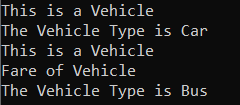##### Multipath Inheritance in C++:

A derived class with two base classes and these two base classes has one common base class is called multipath inheritance. Ambiguity can arise in this type of inheritance. For a better understanding, please have a look at the below image.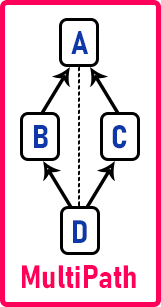Suppose we have one method that is function Fun is present in class A. Then this function will be available via class B and via class C in the class D. So, when you call the function, it means there are two copies of functions in the D that are coming from B and C. This is called a multipath inheritance.

##### Example to Understand Multipath Inheritance in C++:
```#include <iostream>
using namespace std;

class A
{
public:
void Fun()
{
cout << "This is Class A Fun Function\n";
}
};

class B:public A
{
public:
B()
{
cout << "B Class Constructor Executed\n";
}
};

class C:public A
{
public:
C()
{
cout << "C Class Constructor Executed\n";
}
};

class D:public B, public C
{
public:
D()
{
cout << "D Class Constructor Executed\n";
}
};

// main function
int main()
{
D obj1;
obj1.Fun();
return 0;
}
```
###### Output: Error:

“D::Fun” is ambiguous
ambiguous access of ‘Fun’

So, the function Fun can be called in D via B or via C. So here we get the ambiguity.

##### How to Remove Ambiguity Problem in C++?

There are two ways to avoid the Ambiguity Problem in C++. They are as follows:

1. Avoiding Ambiguity using Scope Resolution Operator
2. Avoiding Ambiguity using the virtual Base Class
##### Avoiding Ambiguity Problem in C++ using Scope Resolution Operator

Using the Scope Resolution Operator (::) we can manually specify the path from where the function will be accessed which is shown in the below example.

```#include <iostream>
using namespace std;

class A
{
public:
void Fun()
{
cout << "This is Class A Fun Function\n";
}
};

class B:public A
{
public:
B()
{
cout << "B Class Constructor Executed\n";
}
};

class C:public A
{
public:
C()
{
cout << "C Class Constructor Executed\n";
}
};

class D:public B, public C
{
public:
D()
{
cout << "D Class Constructor Executed\n";
}
};

int main()
{
D obj1;
obj1.B::Fun();
obj1.C::Fun();
return 0;
}
```
###### Output: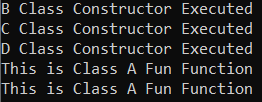##### Avoiding Ambiguity using Virtual Base Class in C++

Suppose we have a class X with some code as follows:
class A {
—-;
}
Then we have another class B which is publicly inheriting from A as follows:
class B : virtual public A{
—–;
}
Then we have another class C which is publicly inheriting from A as follows:
class C : virtual public A{
—–;
}
So now these two classes B and C are called virtual base classes. Now if we have a class D which is inheriting from B and C as follows, then we will not get the ambiguity problem.
class D : public B, public C{
—–;
}
As we have written virtual at the time of inheriting then the problem of ambiguity of that function appearing via B and C will be removed. So that’s it.

##### Example to Resolve Ambiguity Problem using Virtual Base Classes in C++
```#include <iostream>
using namespace std;

class A
{
public:
void Fun()
{
cout << "This is Class A Fun Function\n";
}
};

class B:virtual public A
{
public:
B()
{
cout << "B Class Constructor Executed\n";
}
};

class C:virtual public A
{
public:
C()
{
cout << "C Class Constructor Executed\n";
}
};

class D:public B, public C
{
public:
D()
{
cout << "D Class Constructor Executed\n";
}
};

int main()
{
D obj1;
obj1.Fun ();
return 0;
}
```
###### Output: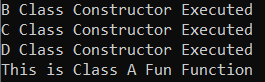Basically, from the C++ compiler, we have limited built-in classes available. If you are developing your own C++ classes for your project and if you are organizing them in the hierarchy, you are inheriting them from one another then there may be a chance that you may come across this type of situation. So, to avoid that situation you can make the base classes as virtual base classes. They are useful for removing the ambiguity of the features of the parent class in their derived class that is coming via multiple paths.

1.